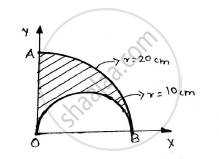Advertisement Remove all ads

# Find the Co-ordinate of the Centroid of the Area as Shown in the Given Figure. - Engineering Mechanics

Answer in Brief

Find the co-ordinate of the centroid of the area as shown in the given figure.Advertisement Remove all ads

#### Solution

 Figure Area(mm2) X co-ordinate of centroid (mm) Y co-ordinate of centroid (mm) Ax(mm2) Ay(mm2) Quarter circle 0.25 x π x R2=0.25 x 202 x 𝝅=314.1593 (4R)/(3pi)=(4xx20)/(3pi)=8.4883 (4R)/(3pi)=(4xx20)/(3pi)=8.4883 2666.6667 2666.6667 Semi-circle(to be removed) -0.5 x π x r2= -157.0796 10 (4R)/(3pi)=(4xx10)/(3pi)=4.2441 -1570.7963 -666.6667 Total 157.0796 1095.8704 2000

∴ X co-ordinate of centroid (x̅)= (∑ Ax)/(∑A)=(1095.8704)/(157.0796)=6.9765 cm

∴ Y co-ordinate of centroid (y)=(∑ AY)/(∑A)=(2000)/(157.0796)=12.7324 cm
Centroid = (6.9765,12.7324) cm

Concept: Velocity and Acceleration in Terms of Rectangular Co-ordinate System
Is there an error in this question or solution?
Advertisement Remove all ads

#### APPEARS IN

Advertisement Remove all ads
Advertisement Remove all ads
Share
Notifications

View all notifications

Forgot password?